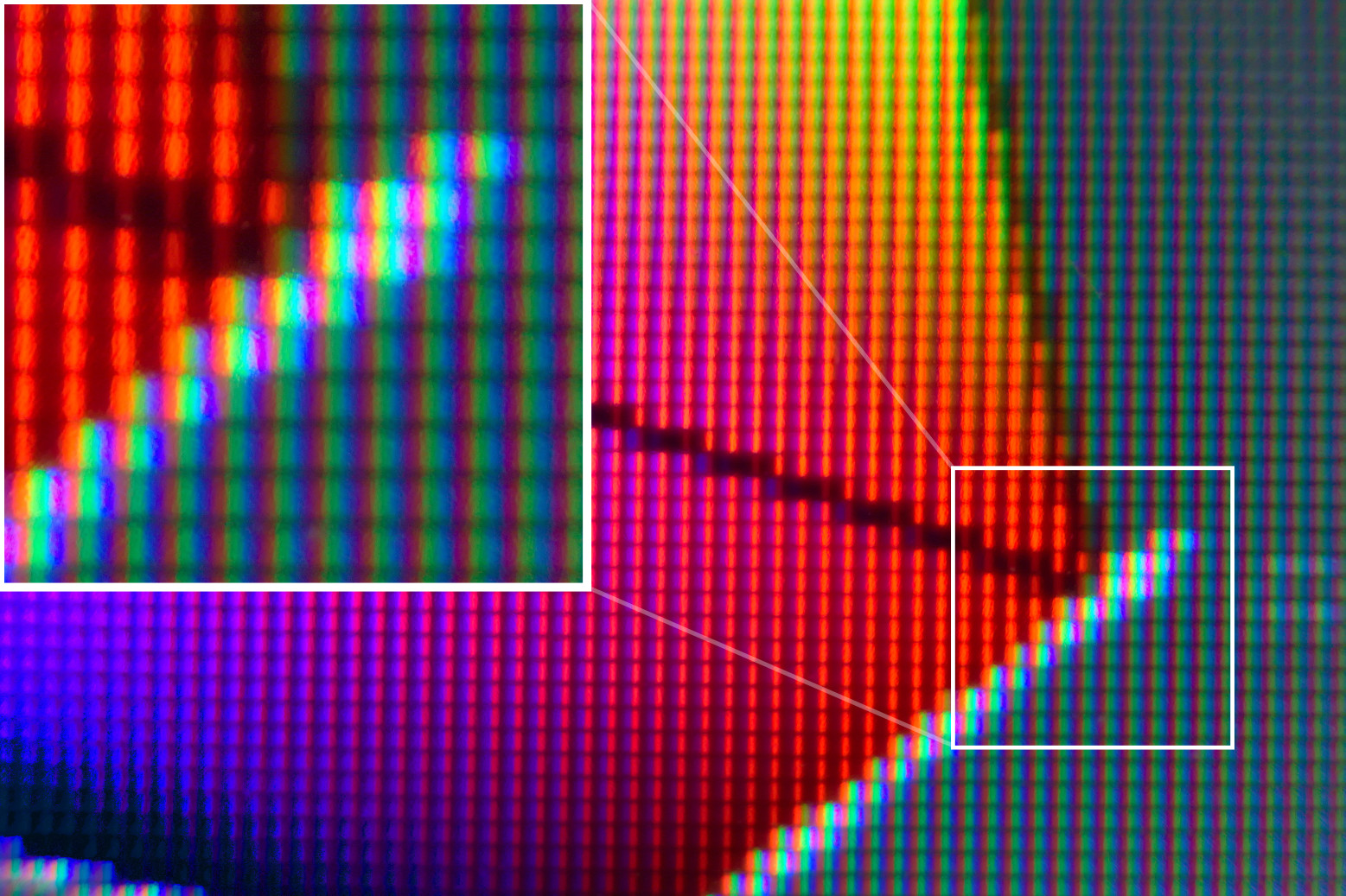# RGB

RGB is an acronym for red, green, and blue. Colors in this system are produced by adding different intensities of red, green and blue light. This principle is called additive color mixing and is used e.g. in LCD displays as shown in the figure below. Each pixel is made up of a red, a green, and a blue subpixel.Close-up photograph of a matte LCD monitor.

## The RGB Color Model

The RGB color model can be understood as a cube in a three-dimensional Cartesian coordinate system with one axis each for the red, green and blue channels [BB09b, p. 186]. A color can therefore be defined as a vector $$(r,g,b)^\mathsf{T}$$, where $$r$$, $$g$$, and $$b$$ are the color's intensities for each channel. For the purpose of distinguishing RGB from other color models, we define the tuple $$(r, g, b)_\text{RGB} := (r,g,b)^\mathsf{T}$$. The figure shows the RGB cube with each channel's value in the $$[0, 1]$$ interval. However, in computer graphics, integral values between 0 and 255 – between 0 and FF in the hexadecimal system – are also a popular choice. For example, RGB colors can be specified in either notation both in CSS [Çe11] and OpenGL.The RGB cube.
Click and drag to rotate, scroll to zoom. On a touch screen, drag to rotate around the y-axis and use two fingers to zoom or to rotate around the x-axis.